## Friday, September 23, 2005

### Joseph-Louis Lagrange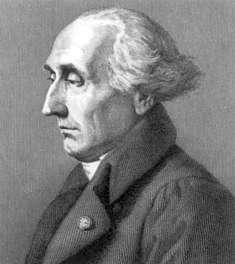Joseph-Louis Lagrange was born in Turin, Italy on January 25, 1736. His original name was Giuseppe Luigi Lagrangia. He would later change his name as he made Prussia and France his home.

His father was a well-to-do mechant who worked for the King of Sardinia. Unfortunately, due to speculations, his father lost much of his wealth. Lagrange went to the college of Turin. He became interested in mathematics when he was 17 and he discovered a paper by Edmund Halley. Within one year, he had impressed the college with his skills in mathematics and became a lecturer at the artillery school.

When Lagrange was 19, he sent a letter to the famous mathematician Leonhard Euler describing a solution to the isoperimetrical problem. Euler was very impressed with Lagrange's method and conceded that it was superior to his own. Euler had been planning to publish his solution to the problem but after viewing Lagrange's solution, he withdrew his paper and let Lagrange publish the first solution to the problem. This paper helped to establish Lagrange as one of the top mathematicians of his time. The solution would serve as the foundation for what became known as the calculus of variations.

In 1758, Lagrange organized the Turin Academy which was a mathematical society that focused on mathematics. This group would go on to publish 5 volumes of mathematics. These volumes presented very high quality mathematics including topics such as a theory of the propagation of sound, a general differential equation for motion, a complete solution to an equation for a string vibrating traversely, recurring series, probabilities, more details on the calculus of variations, the principle of least action, integral calculus, number theory, and general differential equations for the motion of three bodies. By 1761, Lagrange had established himself as one of the greatest mathematician living. In 1764, he began his study of the libations of the moon and published his mathematical explanation for why the moon only displays the same face to the earth.

In 1766, Euler left Berlin. Frederick the Great invited Lagrange to come fill his post. Frederick wrote that the "greatest king in Europe" would like to have the "greatest mathematician of Europe" as resident in his royal court. Lagrange accepted his invitation and would stay in Prussia for the next 20 years.

While in Prussia, he wrote his famous Mecanique Analytique. His productivity at this point was amazing. He was producing a very high quality mathematical paper at the rate of one per month. He would spend his time thinking through each of his ideas and only when he had thought his ideas through did he write his papers. It is said that he was able to write out his papers complete without a single correction required. Topics included astronomical observations, pressure exerted by fluids in motion, integration by infinite series, a paper on the Jovian system, an essay on the problem of three bodies, secular equations of the moon, and cometary pertubations. In the course of these papers, he won many of the prizes (1772, 1774, and 1780) that were offered by the French Academy. Between 1772 and 1788, he worked to simplify Newton's equations. He reformulated Newtonian mechanics creating what is today known as Lagrangian mechanics.

In 1787, Frederick died and Lagrange accepted the offer of Louis XVI to move to Paris. He turned down invitations from Spain and Naples. He set up in residence in one of the special apartments of the Louvre. In 1792, he married for the second time.

The Reign of Terror began in 1793. In September 1793, an order was placed requiring all foreigners to leave France. He was able to get an exemption to this order based on the help of Antoine Lavoisier. Later, in 1798, his friend Lavoisier, after a trial that lasted only a day, was put to death by guillotine.

Lagrange wrote of his friend:
It took only a moment to cause this head to fall and a hundred years will not suffice to produce its like.
In 1797, he became a professor of the Polytechnique Ecole in Paris. His lectures at the Polytechnique became very important and influential mathematical papers. It was here that he did his work on Continued Fractions and his mentorship of Sophie Germain.

Lagrange was still working on a revision of his Mecanique Analytique when he died in 1813. His achievements are astounding. He is one of the most prolific and important mathematicians of all time.

References

## Wednesday, September 21, 2005

### Binomial Theorem

The Binomial Theorem was first presented by Sir Isaac Newton. He came up with this principle based on the work done by Blaise Pascal (Pascal's Triangle) and Pierre de Fermat. Newton's result is actually deeper than the one presented in today's blog. He was able to generalize the Binomial Theorem to include exponents that could be any real number. When the Binomial Theorem is presented in this context, it is often refered to as the Binomial Series.

The proof presented here relies on the Principle of Mathematical Induction. If you are not familiar with Mathematical Induction, then you may wish to start here.

The proof is based on factorials. Importantly, it relies on the following definition: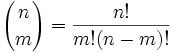With this definition in mind, let's take a look at the proof for the Binomial Theorem:

Theorem: Binomial Theorem(1) The assumption here is that n ≥ 2. For n=1, we know that (a+b)1 = a1 + b1 = a + b.

(2) Let S be the set of all numbers n ≥ 2 that supports the binomial theorem. If there are no such numbers, then S would be empty.

(3) We know that 2 is an element of S since:

(a) (a + b)2 = a2 + 2ab + b2 (See here for details if needed)

(b)= a2 + 2ab + b2

(4) So, let us suppose that there is a value n such that 2 thru n, the binomial theorem holds. If the binomial theorem, for example, didn't hold for 3, then n would equal 2.

(5) Now, we note that:
(a + b)n+1 = (a + b)n(a + b) = a(a + b)n + b(a + b)n

(6) Now, by (4), we have: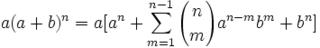and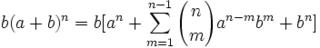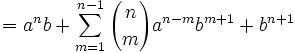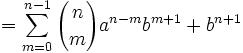(7) The trick comes down to realizing that:(a) We notice that m=0 thru n-1 and m=1 thru n contains the same number of elements.

(b) At each point, m as a value of the factorial expression is the same. In the case of m = 0, the first element is 0, the second element is 1, and so on until we get to n-1. In the case of m=1, the first element is 0, the second element is 1, and so on until we get to n.

(c) At each point, the exponent for a is the same. In the case of m=0, the first exponent is n-m = n, the second coefficient has a power of n-1, followed by n-2, and so on until we get to n-(n-1) = 1. In the case of m=1, the first exponent is n-m+1=n, the second coefficient has a power of n-1 and so on until we get to the last one which is n-n+1 which is equal to 1.

(d) Finally, at each point, the exponent for b is the same. In the case of m=0, the first power of b is 1, followed by 2, and so on until we get to n-1+1 which = n. In the case of m=1, the first power of b is 1, followed by 2, and so until we get to the end which b to the power of n.

(8) Applying our results from (6) and (7) and applying Pascal's Rule, we note that: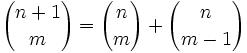Which gives us that: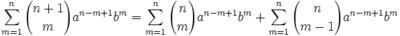(9) And putting this all together, gives us: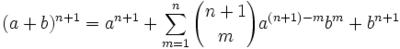QED

## Tuesday, September 20, 2005

### Pascal's Triangle and Pascal's Rule

Before we can proceed to the Binomial Theorem, we need to review a lemma that was introduced in the west by Blaise Pascal and which is today known as Pascal's Rule. The rule itself stems from an analysis of Pascal's Triangle.

Pascal's rule requires a basic understanding of the mathematics of permutations which concerns the problem of counting the number of different selections that can be made from a given set. For those who would like a review of the basic ideas of permutations and factorials, please start here. Some examples are below.

If we have only 1 element, then there is only 1 way of selecting 1 element. But if we have 2 elements, we have 2 different ways to select 1 element. If we have 15 elements, then there are 15 different ways to select 1 element and, it turns out, (15*14)/2 ways to select 2 elements. In other words, if we have n total elements and we want to select m of them, there are exactly n!/[m!(n-m)!] ways to select them where order doesn't matter. (See here for further explanation)

The formula for counting the number of ways to select m elements from a set of n total elements can be characterized by this shorthand notation: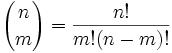In the case of selecting 2 items out of 15, we get the following: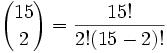Which gives us: 15!/(2!)(13)! = (15*14)/2.

With these ideas in mind, we can proceed to look at Pascal's Triangle. Pascal's Triangle is a way to characterize exponents such as (a + b)n

For example, the Triangle starts out as follows:

(n=0) 1
(n=1) 1 1
(n=2) 1 2 1
(n=3) 1 3 3 1
(n=4) 1 4 6 4 1
...

In each case, the number in the next row is equal to the sum of the two numbers above it (and if only one number is above it, then it is equal to this one number). We can keep on going as long as we like.

Let's see how this maps into the equation (a+b)n

(a + b)0 = (1)1
(a + b)1 = (1)a +(1) b
(a + b)2 = (1)a2 + (2)ab + (1)b2
(a + b)3 = (1)a3 + (3)a2b + (3)ab2 + (1)b3
(a + b)4 = (1)a4 + (4)a3b + (6)a2b2 + (4)ab3 + (1)b4

It turns out that each element of Pascal's Triangle can be built from an equation using the above equation. If we remember that 0! = 1, then we get the following

For n = 0, the single element is equal to: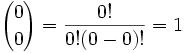For n = 1, each element can be described by the notation below: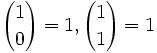For n = 2, each element can be described by:For n = 3, each element can be described by: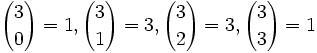And so on.

This is useful because it allows to pick any exponent n and generate the list of coefficients that make up its values.

For example, we know that the coefficients for n=7 will be:So far so good. But wait a minute. When we were creating the triangle from step 1, we were adding each coefficient from the previous values. Is there a relationship here that can be captured in terms of the equation?

This is Pascal's Rule which is presented below.

Lemma: Pascal's Rule: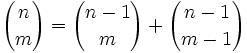(1)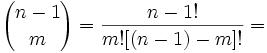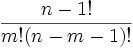(2)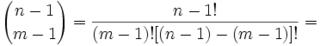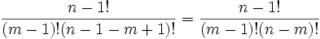(3) Now, the trick is to be able to add both of these values as fractions. This can be done based on the following equalities:

m! = (m)(m-1)!
(n-m)! = (n-m)(n-m-1)!

(4) Applying (3) to (1) and (2) gives us: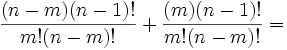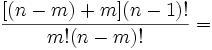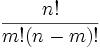QED